#### 期刊菜单

Study on Adsorption of Europium Ion by Magnesium Hydroxide
DOI: 10.12677/HJCET.2021.113023, PDF, HTML, XML, 下载: 176  浏览: 252

Abstract: Due to the unique physical and chemical properties, rare earth elements have been widely used in new energy, new materials, aerospace and other fields. Rare earth elements have been called “modern industrial vitamins” and become an indispensable part of modern industry. The effects of various adsorption factors on the adsorption capacity of europium ion were investigated by magnesium hydroxide adsorption. The experimental results show that the adsorption capacity of magnesium hydroxide on europium ion is up to 1650 mg/g and the removal rate of europium ion in the solution is about 91% when the initial concentration is 600 mg/L, the solid-liquid ratio is 1:3, the pH is 6 and the adsorption temperature is 25˚C. For europium ion solution with initial concentration less than 500 mg/L, the removal efficiency of magnesium hydroxide can reach more than 98%.

1. 引言

2. 材料与仪器

2.1. 实验试剂

2.2. 实验仪器

SP-752PC紫外分光光度计(上海光谱仪器有限公司)；DF-101S集热式恒温水浴锅(巩义予华仪器有限责任公司)；85-1A型磁力搅拌器(上海梅颖浦仪器仪表制造有限公司)；PHB-5笔式pH计(杭州奥立龙仪器有限公司)。

3. 实验方法

3.1. 吸附实验

${q}_{t}=\frac{\left({C}_{0}-{C}_{t}\right)×V}{m}$ (1)

${q}_{e}=\frac{\left({C}_{0}-{C}_{e}\right)×V}{m}$ (2)

$\text{A%}=\frac{\left({C}_{0}-{C}_{e}\right)}{{C}_{0}}×100\text{%}$ (3)

3.2. 动力学热力学模拟

1) 热力学模拟

${K}_{d}=\frac{{C}_{0}-{C}_{e}}{{C}_{e}}×\frac{V}{m}$ (4)

$\Delta {G}^{\theta }=-RT\mathrm{ln}{K}_{d}$ (5)

$\Delta {G}^{\theta }=\Delta {H}^{\theta }-T\Delta {S}^{\theta }$ (6)

$\mathrm{ln}{K}_{d}=\frac{\Delta {S}^{\theta }}{R}-\frac{\Delta {H}^{\theta }}{RT}$ (7)

2) 动力学模拟

4. 结果分析与讨论

4.1. pH对吸附效果的影响

pH对铕离子的吸附效果如图1所示，当pH < 4.5时，吸附效果很差，此时溶液中铕元素全是以Eu3+离子形式存在，不利于与氢氧化镁形成化学键，且当溶液处于强酸性条件下时，溶液中存在大量的H+，易于氢氧化镁反应从而阻碍铕离子的吸附。而当pH > 6时，铕元素在溶液中以EuOH2+、Eu(OH2)+、Eu(OH)3 (aq)和 $\text{Eu}{\left(\text{OH}\right)}_{4}^{-}$ 的结构存在 ，更易与氢氧化镁形成氢键利于吸附，故本实验的pH控制在6为最佳。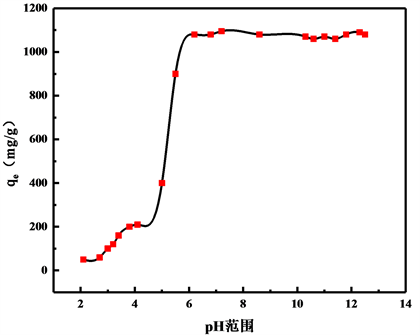Figure 1. Influence of pH on adsorption effect

4.2. 温度对吸附效果的影响及热力学计算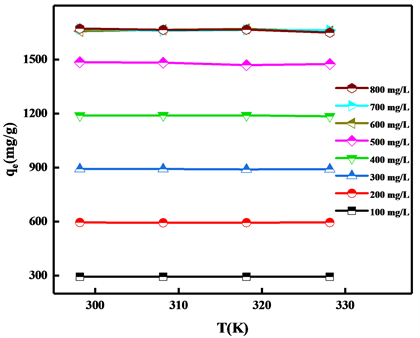Figure 2. Influence of temperature on adsorption effect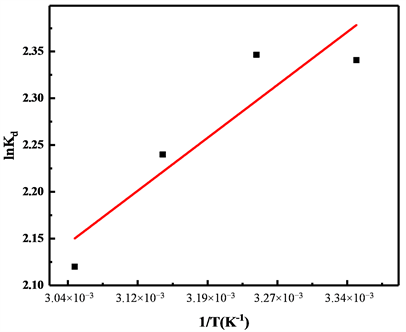Figure 3. The relationship between lnKd and1/TTable 1. Thermodynamic constant for the adsorption of Eu3+ by magnesium hydroxide

4.3. 吸附时间对吸附效果的影响及动力学模拟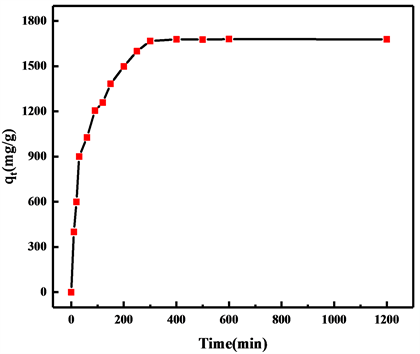Figure 4. Effect of time on adsorption capacity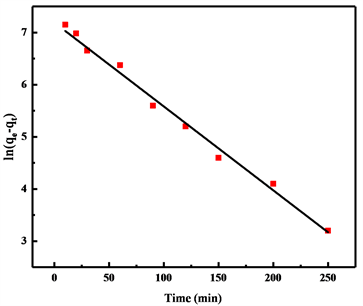(a)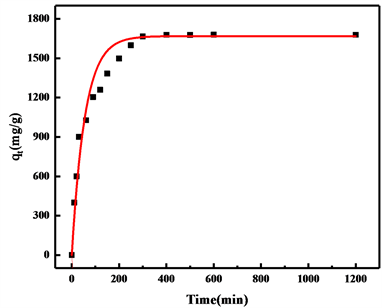(b)(c)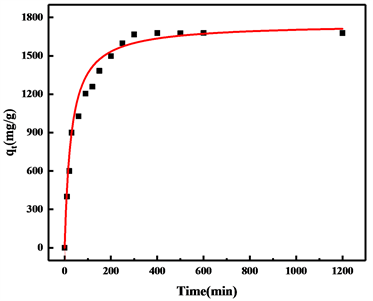(d)

Figure 5. (a) (b) Linear and nonlinear of the pseudo-first-order kinetic models; (c) (d) Linear and nonlinear of the pseudo-second-order kinetic modelsTable 2. The pseudo-first-order and pseudo-second-order kinetic model constants for adsorption of Eu3+

4.4. 吸附前后表征分析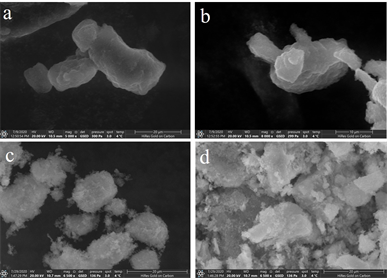Figure 6. The SEM images (a) (b) before the adsorption; (c) (d) after the adsorption

5. 结论

NOTES

*通讯作者。

  刘晓杰, 许涛, 郝茜, 崔建国. 钕铁硼磁性材料生产工艺及其废料综合利用的研究进展[J]. 稀有金属与硬质合金, 2014. 42(3): 48-53.  Tao, X.U. and Peng, H.Q. (2009) Formation Cause, Composition Analysis and Comprehensive Utilization of Rare Earth Solid Wastes. Journal of Rare Earths, 27, 1096-1102. https://doi.org/10.1016/S1002-0721(08)60394-4  Jowitt, S.M., et al. (2018) Recycling of the Rare Earth Elements. Current Opinion in Green and Sustainable Chemistry, 13, 1-7. https://doi.org/10.1016/j.cogsc.2018.02.008  谷静维. 纳米氢氧化镁的制备及其性能研究[D]: [硕士学位论文]. 郑州: 郑州大学, 2014.  Yan, T., Luo, X., Zou, Z., et al. (2017) Adsorption of Uranium (VI) from a Simulated Saline Solution by Alkali-Activated Leather Waste. Industrial & Engineering Chemistry Research, 56, 3251-3258. https://doi.org/10.1021/acs.iecr.6b04425  Yuh-Shan, H. (2004) Citation Review of Lagergren Kinetic Rate Equation on Adsorption Reactions. Scientometrics, 59, 171-177. https://doi.org/10.1023/B:SCIE.0000013305.99473.cf  Wang, Y.Q., Fan, Q.H., Li, P., et al. (2011) The Sorption of Eu(III) on Calcareous Soil: Effects of pH, Ionic Strength, Temperature, Foreign Ions and Humic Acid. Journal of Radioanalytical and Nuclear Chemistry, 287, 231-237. https://doi.org/10.1007/s10967-010-0809-9